## Saturday, July 30, 2016

### On 2D Ordered HWT Coefficients of Diagonal Lines

Problem

Suppose that an image has diagonal lines. What happens when a 2D ordered Haar Wavelet Transform (HWT) applied to it? For the sake of clarity and simplicity, let us assume that an image contains only 1 diagonal line at different location. The second simplifying assumption is that an image is n x n where n is an integral power of 2. This is a requirement for 2D HWT. If the size of the image is not n x n, the image can typically be reduced or padded. The third simplifying assumption is that the thickness of a line is equal to exactly 1 pixel. The fourth assumption is that a diagonal line runs from a point in the top left quadrant to a point in the bottom right quadrant or from a point in the top right quadrant to a point in the bottom left quadrant. This post tabulates what happens to the 2D ordered HWT coefficients in some cases. In describing the points, I will use the OpenCV notation where x is the horizontal axis going right from the top left corner and y is the vertical axis going down from the top left corner. In other words, (x, y) describes the point colum x and row y.

Sample Diagonal Lines

Figures below are given in triples, e.g., Figure 1, Figure 2, and Figure 3. The first figure is an 8x8 image with a diagonal line. The second figure is the corresponding pixel value matrix. The third figure is the 2D ordered HWT coefficients after 1 iteration applied to the matrix. The colors in the matrices in the second and third matrices of each triplet denote the 2x2 blocks and the corresponding average, horizontal, vertical, and diagonal coefficients.Figure 1. 8x8 image with a line from (3, 0) to (0, 3)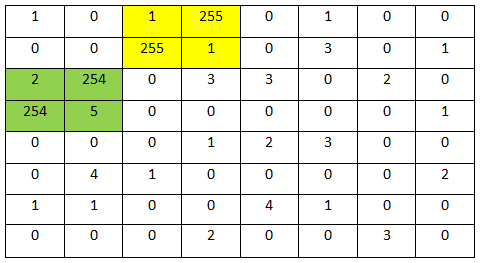Figure 2. Pixel value matrix of image in Fig. 1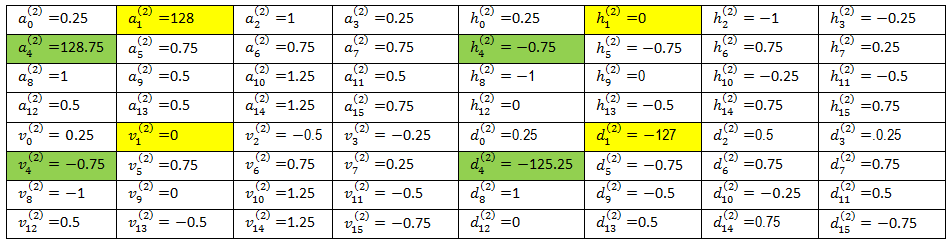Figure 3. 2D ordered HWT coefficients after 1 iteration on matrix in Fig. 2Figure 4. 8x8 grayscale image with a line from (3, 0) to (7, 4)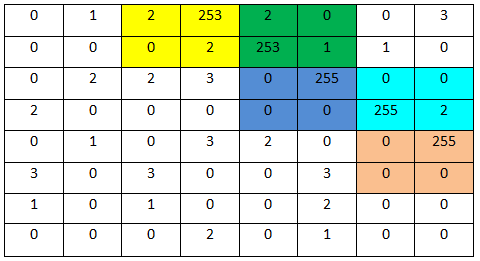Figure 5. Pixel value matrix of image in Fig. 4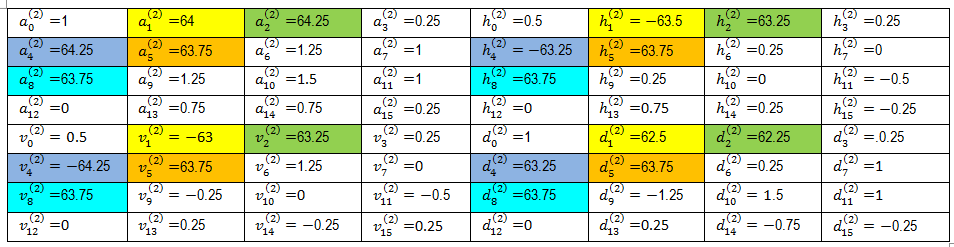Figure 6. 2D ordered HWT coefficients after 1 iteration applied to matrix in Fig. 5Figure 7. 8x8 image with line from (4, 0) to (0, 4)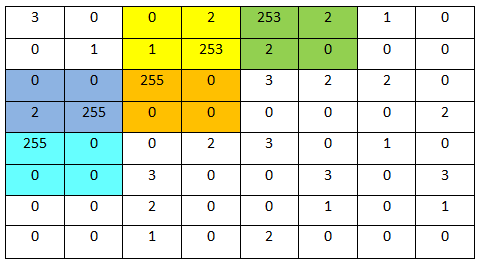Figure 8. Pixel value matrix of image in Fig. 7Figure 9. 2D ordered HWT coefficients after 1 iteration on matrix in Fig. 8

Observations

In each 2x2 block on a diagonal line, the diagonal coefficient is always large in absolute magnitude. The horizontal or vertical coefficients can either be zero (or close to it) or large in absolute magnitude. The values of the horizontal and vertical coefficients depend on where the white pixels are located in 2x2 blocks. If both white pixels are located along either diagonal, the horizontal and vertical coefficients are zero.